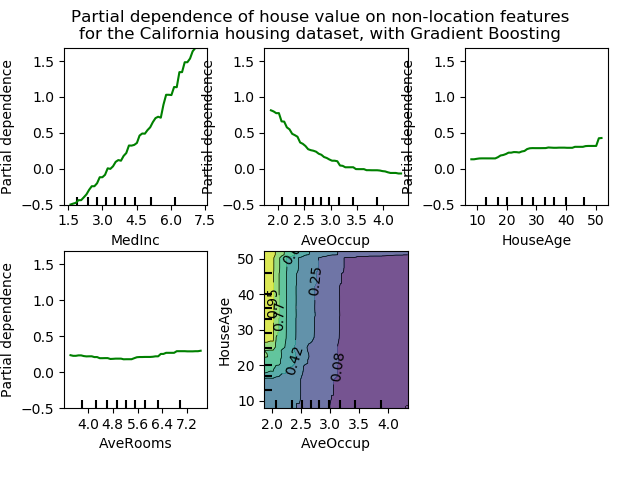# 4.1. 部分依赖图`sklearn.inspection`提供了一个方便的函数`plot_partial_dependency`来创建单向和双向PDP。在下面的示例中，我们展示了如何创建一个局部依赖图的网格:两个用于特征0和1的单向PDP，以及两个特征之间的双向PDP:

``````>>> from sklearn.datasets import make_hastie_10_2
>>> from sklearn.inspection import plot_partial_dependence

>>> X, y = make_hastie_10_2(random_state=0)
...     max_depth=1, random_state=0).fit(X, y)
>>> features = [0, 1, (0, 1)]
>>> plot_partial_dependence(clf, X, features)
``````

``````>>> from sklearn.datasets import load_iris
...     max_depth=1).fit(iris.data, iris.target)
>>> features = [3, 2, (3, 2)]
>>> plot_partial_dependence(mc_clf, X, features, target=0)
``````

``````>>> from sklearn.inspection import partial_dependence

>>> pdp, axes = partial_dependence(clf, X, )
>>> pdp
array([[ 2.466...,  2.466..., ...
>>> axes
[array([-1.624..., -1.592..., ...
``````

　对于分类问题，目标响应可以是类的概率（二元分类的正类）或决策函数。